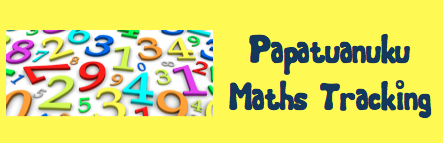I am learning about numbers.

This is how I am getting on…

Emergent - Stage 0*Achieved

KNOWLEDGE

I am learning to...

 Date:            ✓ READ Numbers up to 10   2   two   7   seven COUNT Forwards up to 101   2   3   4   5   ... Backwards from 1010   9   8   7   6   5 ... SAY The number after from 0 - 10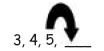The number before from 0 - 10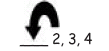ORDER Numbers up to 52   3   5

STRATEGY

I am learning to...

 COUNT The number of objects in a set to 10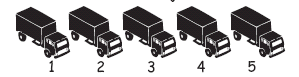GET A set of objects up to 10One to One Counting - Stage 1*Achieved

KNOWLEDGE

I am learning to...

 Date:            ✓ READ Numbers up to 10   3   three   9   nine COUNT Forwards up to 101   2   3   4   5   ... Backwards from 1010   9   8   7   6   5 ... SAY The number after from 0 - 10The number before from 0 - 10ORDER Numbers up to 101   2   4   7   8 KNOW Patterns to 5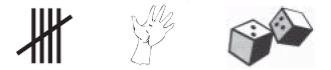STRATEGY

I am learning to...

 JOIN Groups of objects togetherSPLIT A number of objects into groups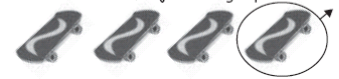Counting from One on Materials - Stage 2*Achieved

KNOWLEDGE

I am learning to...

 Date:           ✓ READ Numbers up to 2014   fourteen ½        ¼ COUNT Forwards up to 20 1   2   3   4   5   6   7   8   9   10   11   ... Backwards from 20 20   19   18   17   16   15   14   13  ... SAY The number after from 1 to 20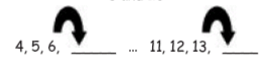The number before from 1 to 20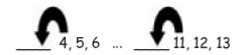ORDER Numbers to 20 4   7   8   13   17   18 KNOW Groups within 5 (basic facts)3 + 1 = __      3 + __ = 44 - 1 = __      4 - __ = 3 Groupings with 5 (basic facts)5 + 3 = __      5 + __ = 8

STRATEGY

I am learning to...

 SOLVE + & - Up to 10 by counting all the objectsCounting from One by Imaging - Stage 3*working at

KNOWLEDGE

I am learning to...

 Date:           ✓ READ Numbers up to 2019   nineteen achieved ½        ¼ achieved COUNT Forwards up to 20 12   13   14   15   ... achieved Backwards from 20 17   16   15   14   13  ... achieved SKIP COUNT Forwards in twos to 20 2   4   6   8   10   12   14   16   18   20 Forwards in fives to 505  10  15  20  25  30  35  40  45  50 Forwards in tens to 10010  20  30  40  50  60  70  80  90  100 SAY The number after from 1 to 2016/2 The number before from 1 to 2016/2 ORDER Numbers to 20 4   7   8   13   17   18 nearly there KNOW Groups that make 107 + 3 = __      7 + __ = 1010 - 7 = __      10 - __ = 3 Not knowledge - uses fingers Doubles to 10 (5 + 5)2 + 2 = 4      5 + 5 = 10 Not knowledge - uses fingers

STRATEGY

I am learning to...

 SOLVE + & - Up to 10 by counting all the objects in my head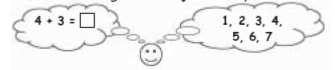9/3 SHARE Objects equally to make ½ and ¼ Share 12 muffins between 4 (¼ of 12)Advanced Counting - Stage 4

KNOWLEDGE

I am learning to...

 Date:           ✓ READ Any number up to 10017   26   38   47   53   74   86   99 ½   ¼   ⅓   ⅕   1/10 COUNT Forwards in ones, twos, fives and tens up to 100 Backwards in ones, twos, fives and tens  from any number before 100 SAY The number after in the range 0 - 100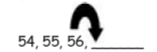The number before in the range 0 - 100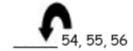ORDER Numbers to 100 … and then 100022   25   34   37   45   73  & then 1000 KNOW Teen facts (ten &)10 + 5 = 15      10 + 8 = 1810 + __ = 19      13 - __ = 10 Tens in decades (how many tens in 60) Tens that add to 100 70 + 30 = 100      40 + __ = 100100 - 20 = __      100 - __ = 10 Doubles to 20 (10 + 10) Halves to 20 (half of 20 = 10)

STRATEGY

I am learning to...

 SOLVE up to 100 by counting on from the biggest number, in my head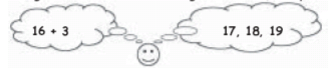within 100 by counting back from the biggest number, in my head x   by skip counting in twos, fives                       and tens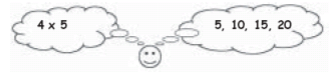FIND Objects equally to make ½ and ¼ Share 12 muffins between 4 (¼ of 12)Early Additive Part/Whole - Stage 5

KNOWLEDGE

I am learning to...

 Date:           ✓ READ Numbers up to 1000333      479      983 ½   ⅓   ¼   ⅕   1/10 COUNT Forwards by ones, tens and hundreds up to 1000 Backwards by ones, tens and hundreds from 1000 SKIP COUNT Forwards and backwards in threes3   6   9   12   15   18   21 ... SAY The number 1, 10 or 100 more than numbers up to 1000 The number 1, 10 or 100 less than any number before 1000 ORDER Numbers to 1000 and then 100001   58   376   837 Fractions with the same denominator¼   2/4  ¾   4/4 ROUND Three digit numbers to the nearest 10 or 100 246 ------> 250 (nearest 10) KNOW Groups that make 2017 + 3 = __      17 + __ = 2020 - 7 = __      20 - __ = 13

STRATEGY

I am learning to...

 SOLVE + problems by Using fives 8 + 7 = (5 + 5) + (3 + 2) SOLVE + and - problems by Using doubles8 + 7 = (8 + 8) - 117 - 8 = (16 - 8) + 1 Making tens 8 + 7 = (8 + 2) + 512 - 6 = (12 - 2) - 4 Making tidy tys 16 + 8 = (16 + 4) + 435 - 9 = (35 - 5) - 4 Using place value 33 + 16 = (30 + 10) + (3 + 6)76 - 42 = (70 - 40) + (6 - 2) SOLVE x problems by Using repeated addition 2 x   -   4 x 2 = (2 + 2 + 2 + 2)3 x   -   5 x 3 = (3 + 3 + 3 + 3 + 3)4 x   -   3 x 4 = (4 + 4 + 4)5 x   -   5 x 5 = (5 + 5 + 5 + 5 + 5)10 x   -  10 x 2 = (10 + 10) FIND A fraction of a number by using repeated addition or subtraction

created by Fiona Hunter,, Russell Street School, Palmerston North

KNOWLEDGE

I am learning to...

 Date:           ✓ READ & ORDER Numbers up to 10000001374      98765      763104 READ Decimals up to three places0.764      0.14      0.8 Symbols for any fraction1 ⅓      ⅘      13/3 ORDER Fractions with different denominators ¼      ⅔      ⅘ SAY The number 1000 more or 1000 less KNOW How many tens and hundreds are in four digit numbers Fractions with the same denominator¼      2/4     ¾ Groups to 1000240 + 760 = 1000 How many twos, threes, fives and tens in numbers up to 100 and any remainders3s in 17 = 5 r 2 ROUND Whole numbers to the nearest 10, 100, and 10005508 ------> 6000 (nearest 1000) Decimals to the nearest whole number 3.49 ------> 4 KNOW Basic facts for subtraction, within 20 Basic facts for multiplication RECORD Results of calculations using empty number lines and written algorithms

STRATEGY

I am learning to...

 SOLVE + and - problems by Using compensation from tidy numbers725 - 389 = (725 - 400) + 11 = 336 SOLVE + and - problems by Using place value 376 + 431 = (300 + 400) + (70 + 30) + (6 + 1) = 801 Using compatible numbers35 + 37 + 65 = (35 + 65) + 37 = 137 Using reversibility 814 - 789 = 789 + __ = 814 Using equal addition72 - 37 = 75 - 40 = 35 Using decomposition 83 - 28 =rename 83 as (70 + 13)70 - 28 = 4242 + 13 = 55 SOLVE x problems by Using doubles 4 x 6 = (2 x 6) x 2 Deriving facts 3 x 6 = (2 x 6) + 6 = 18 Using reversibility 28 ÷ 4 = 4 x __ = 28 Using proportional adjustmentDoubling and halving 3 x 12 = 6 x 6 24 ÷ 4 = 24 ÷ 8 SOLVE PROBLEMS WITH FRACTIONS Mentally using known multiplication and division facts⅓ of 36 = 3 x 12 = 36, so ⅓ of 36 = 12Finding answers to divisions that are fractions using multiplication facts and equal sharing5 ÷ 3 as 1 + ½ + ⅙ (repeated sharing)

created by Fiona Hunter,, Russell Street School, Palmerston North